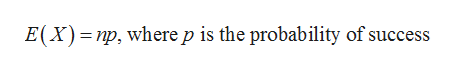For each Bernoulli process, find the expected number of successes: 1) Number of trials =20, Probability of success =0.8   2) Number of trials =210, Probability of success =1/10   3) Number of trials =27, Probability of success =0.2

Question

For each Bernoulli process, find the expected number of successes:

1) Number of trials =20, Probability of success =0.8

2) Number of trials =210, Probability of success =1/10

3) Number of trials =27, Probability of success =0.2

Step 1

From the given information it is needed to calculate the expected number of successes.

The formula for expected number of successes in n trial is:help_outlineImage TranscriptioncloseE(X) np, where p is the probability of success fullscreen
Step 2

In case (1)

n=20 and p=0.8

so,

Step 3

In case (2)

n=210 and p=0.1

so...

Want to see the full answer?

See Solution

Want to see this answer and more?

Our solutions are written by experts, many with advanced degrees, and available 24/7

See Solution
Tagged in

Math